##### Circuit Analysis For Dummies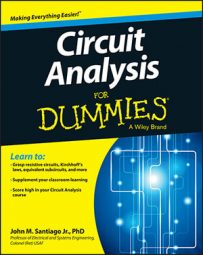Using the Laplace transform as part of your circuit analysis provides you with a prediction of circuit response. Analyze the poles of the Laplace transform to get a general idea of output behavior. Real poles, for instance, indicate exponential output behavior.

Follow these basic steps to analyze a circuit using Laplace techniques:

1. Develop the differential equation in the time-domain using Kirchhoff’s laws and element equations.

2. Apply the Laplace transformation of the differential equation to put the equation in the s-domain.

3. Algebraically solve for the solution, or response transform.

4. Apply the inverse Laplace transformation to produce the solution to the original differential equation described in the time-domain.

To get comfortable with this process, you simply need to practice applying it to different types of circuits such as an RC (resistor-capacitor) circuit, an RL (resistor-inductor) circuit, and an RLC (resistor-inductor-capacitor) circuit.

Consider the simple first-order RC series circuit shown here. To set up the differential equation for this series circuit, you can use Kirchhoff’s voltage law (KVL), which says the sum of the voltage rises and drops around a loop is zero. This circuit has the following KVL equation around the loop:

-vS(t) + vr(t) + vc(t) = 0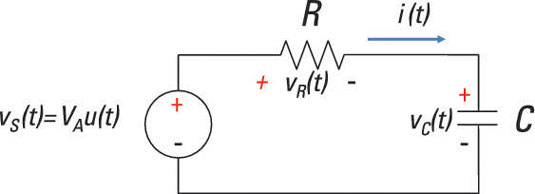Next, formulate the element equation (or i-v characteristic) for each device. The element equation for the source is

vS(t) = VAu(t)
Use Ohm’s law to describe the voltage across the resistor:
vR(t) = i(t)R
The capacitor’s element equation is given as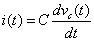Substituting this expression for i(t) into vR(t) gives you the following expression: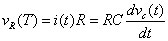Substituting vR(t), vC(t), and vS(t) into the KVL equation leads to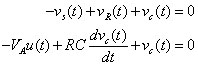Now rearrange the equation to get the desired first-order differential equation: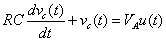Now you’re ready to apply the Laplace transformation of the differential equation in the s-domain. The result is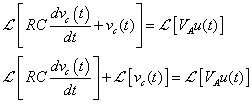On the left, the linearity property was used to take the Laplace transform of each term. For the first term on the left side of the equation, you use the differentiation property, which gives you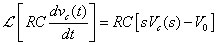This equation uses VC(s) = ℒ[vC(t)], and V0 is the initial voltage across the capacitor.

Using the following table, the Laplace transform of a step function provides you with this: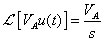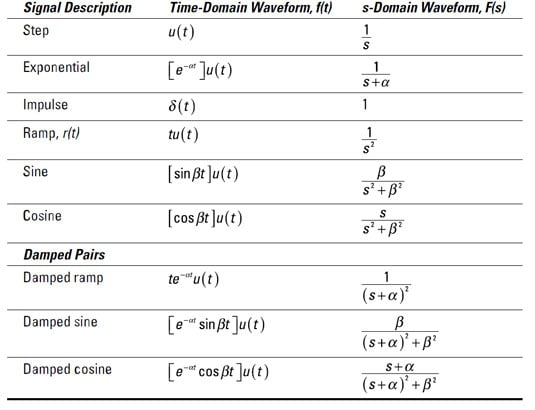Based on the preceding expressions for the Laplace transforms, the differential equation becomes the following: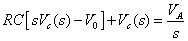Next, rearrange the equation: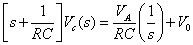Solve for the output Vc(s) to get the following transform solution: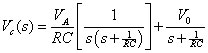By performing an inverse Laplace transform of VC(s) for a given initial condition, this equation leads to the solution vC(t) of the original first-order differential equation.

On to Step 3 of the process. To get the time-domain solution vC(t), you need to do a partial fraction expansion for the first term on the right side of the preceding equation: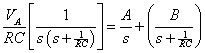You need to determine constants A and B. To simplify the preceding equation, multiply both sides by s(s + 1/RC) to get rid of the denominators: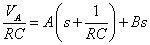Algebraically rearrange the equation by collecting like terms: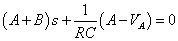In order for the left side of the preceding equation to be zero, the coefficients must be zero (A + B = 0 and A – VA = 0). For constants A and B, you wind up with A = VA and B = –VA. Substitute these values into the following equation: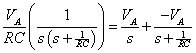Now substitute the preceding expression into the VC(s) equation to get the transform solution: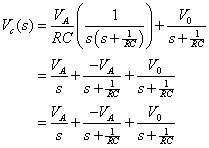That completes the partial fraction expansion. You can then use the table given earlier to find the inverse Laplace transform for each term on the right side of the preceding equation.

The first term has the form of a step function, and the last two terms have the form of an exponential, so the inverse Laplace transform of the preceding equation leads you to the following solution vC(t) in the time-domain: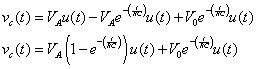The result shows as time t approaches infinity, the capacitor charges to the value of the input VA. Also, the initial voltage of the capacitor eventually dies out to zero after a long period of time (about 5 time constants, RC).